munsell2rgb {aqp} R Documentation

## Convert Munsell Notation to and from RGB color coordinates

### Description

Color conversion based on a look-up table of common soil colors.

### Usage

```munsell2rgb(the_hue, the_value, the_chroma, alpha=1,
maxColorValue=1, return_triplets=FALSE)
rgb2munsell(color)
```

### Arguments

 `the_hue` a vector of one or more more hues, upper-case `the_value` a vector of one or more values `the_chroma` a vector of one or more chromas `alpha` alpha channel value (for transparency effects) `maxColorValue` maximum RGB color value (see `rgb)` `return_triplets` should the function return raw RGB triplets instead of an R color `color` a `data.frame` or `matrix` object containing color-space coordinates: [R, G, B]

### Details

These functions generalizes to vectorized usage, as long as the length of each argument is the same. Both functions will pad output with NA if there are any NA present in the inputs.

### Value

For Munsell to RGB conversion, a vector of R colors is returned that is the same length as the input data. If `return_triplets` is `TRUE`, then a dataframe (of sample length as input) of r,g,b values is returned.

For RGB to Munsell conversion, a dataframe (NA-padded) of hue, value, chroma, and Euclidean distance to nearest matching color is returned.

### Warning

As of `plyr` 1.6 (CRAN), there are cases when this function will fail (https://github.com/hadley/plyr/issues/43). The next version of `plyr` should address this problem. Until then, be sure not to pass in Munsell (hue, value, chroma) as factors.

### Note

Care should be taken when using the resulting RGB values; they are close to their Munsell counterparts, but will vary based on your monitor and ambient lighting conditions. Also, the value used for `maxColorValue` will affect the brightness of the colors. Th default value (1) will usually give acceptable results, but can be adjusted to force the colors closer to what the user thinks they should look like.

### Author(s)

Dylan E. Beaudette

### Examples

``````# Munsell to RGB triplets:
# function is vectorized as long as arguments are the same length
color <- munsell2rgb(the_hue=c('10YR', '2.5YR'), the_value=c(3, 5),
the_chroma=c(5, 6), return_triplets=TRUE)

# RGB triplets to closest Munsell color (in RGB space)
# function is vectorized
rgb2munsell(color)
``````
``````##     hue value chroma sigma
## 1  10YR     3      5     0
## 2 2.5YR     5      6     0
``````
``````# neutral colors map to RGB{0.2, 0.2, 0.2}
munsell2rgb('N', 4, 0)
``````
``````##  "#333333FF"
``````
``````# basic example: no factors!
d <- expand.grid(hue='10YR', value=2:8, chroma=1:8, stringsAsFactors=FALSE)
d\$color <- with(d, munsell2rgb(hue, value, chroma))

# similar to the 10YR color book page
plot(value ~ chroma, data=d, col=d\$color, pch=15, cex=3)
``````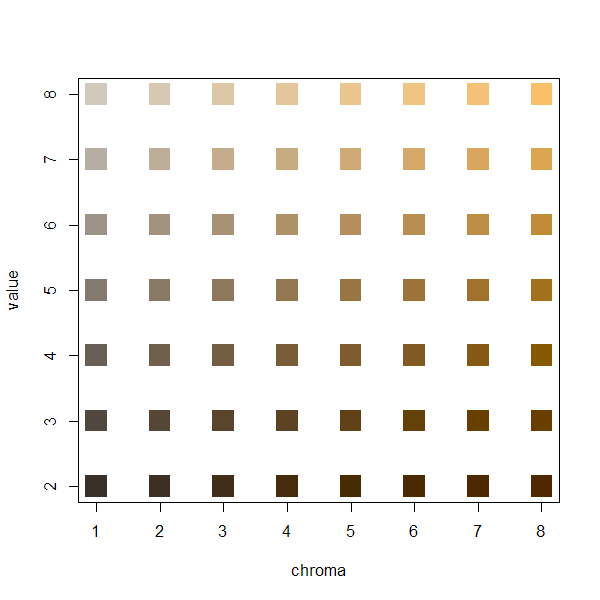``````# multiple pages of hue:
hues <- c('2.5YR','5YR','7.5YR','10YR')
d <- expand.grid(hue=hues, value=2:8, chroma=seq(2,8,by=2), stringsAsFactors=FALSE)
d\$color <- with(d, munsell2rgb(hue, value, chroma))

# plot: note that we are setting panel order from red-->yellow
library(lattice)
xyplot(value ~ factor(chroma) | factor(hue, levels=hues),
main="Common Soil Colors", layout=c(4,1), scales=list(alternating=1),
strip=strip.custom(bg=grey(0.85)),
data=d, as.table=TRUE, subscripts=TRUE, xlab='Chroma', ylab='Value',
panel=function(x, y, subscripts, ...)
{
panel.xyplot(x, y, pch=15, cex=4, col=d\$color[subscripts])
}
)
``````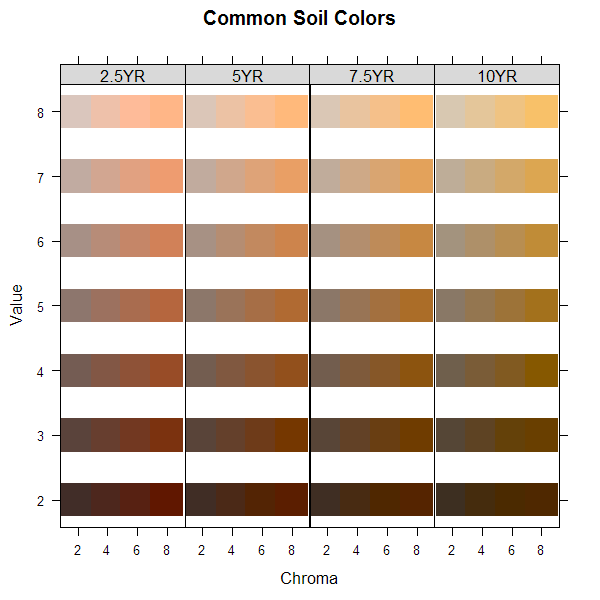``````# try again, this time annotate with LAB coordinates:
if(require(colorspace)) {
d.rgb <- with(d, munsell2rgb(hue, value, chroma, return_triplets=TRUE))
d.lab <- as(with(d.rgb, RGB(r,g,b)), 'LAB')
d <- data.frame(d, d.lab@coords)

xyplot(value ~ factor(chroma) | factor(hue, levels=hues),
main="Common Soil Colors - Annotated with LAB Coordinates", layout=c(4,1),
scales=list(alternating=1), strip=strip.custom(bg=grey(0.85)),
data=d, as.table=TRUE, subscripts=TRUE, xlab='Chroma', ylab='Value',
panel=function(x, y, subscripts, ...) {
panel.xyplot(x, y, pch=15, cex=7, col=d\$color[subscripts])
lab.text <- with(d[subscripts, ], paste(round(L), round(A), round(B), sep='\n'))
panel.text(x, y, labels=lab.text, cex=0.75, col='white', font=2)
}
)

# also demonstrate A ~ hue for each slice of chroma
xyplot(A ~ factor(hue, levels=hues) | factor(value), groups=chroma, data=d,
scales=list(alternating=1), strip=strip.custom(bg=grey(0.85)),
main="A-coordinate vs. Munsell Hue", sub='panels are Munsell value, colors are Munsell chroma',
xlab='Munsell Hue', ylab='A-coordinate', pch=16,
type='b', as.table=TRUE, auto.key=list(lines=TRUE, points=FALSE, columns=4))

}
``````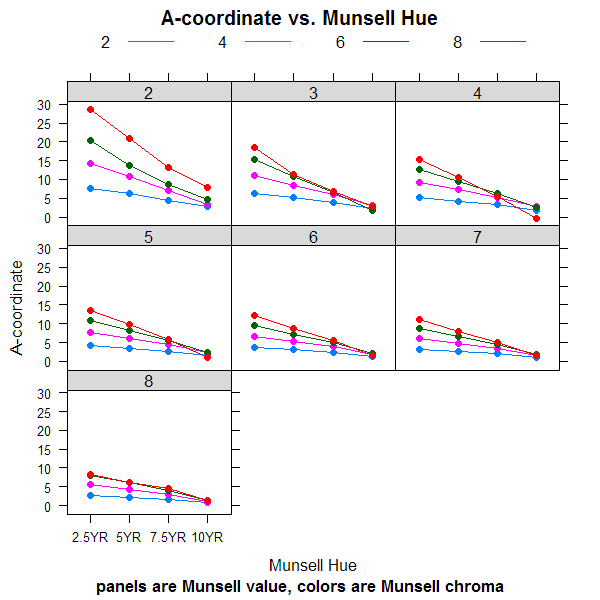``````# soils example
data(sp1)

# convert colors
sp1\$soil_color <- with(sp1, munsell2rgb(hue, value, chroma))

# simple plot, may need to tweak gamma-correction...
image(matrix(1:nrow(sp1)), axes=FALSE, col=sp1\$soil_color, main='Soil Colors')
``````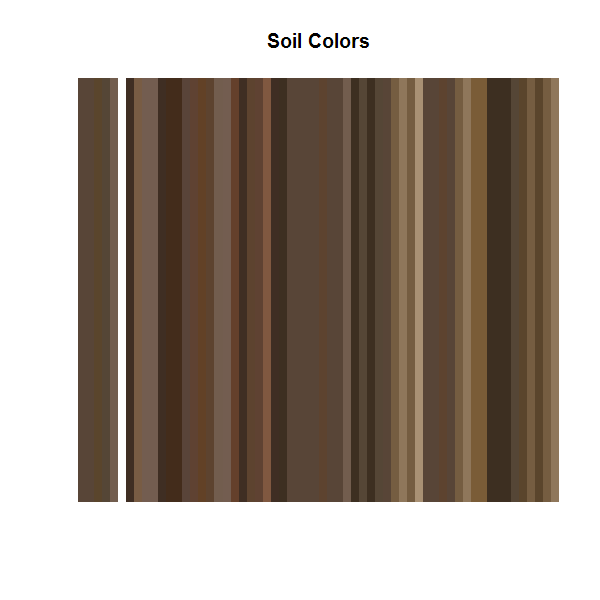``````# convert into a more useful color space
# you will need the colorspace package for this to work
if(require(colorspace)) {
# keep RGB triplets from conversion
sp1.rgb <- with(sp1, munsell2rgb(hue, value, chroma, return_triplets=TRUE))

# convert into LAB color space
sp1.lab <- as(with(sp1.rgb, RGB(r,g,b)), 'LAB')
plot(sp1.lab)
}
``````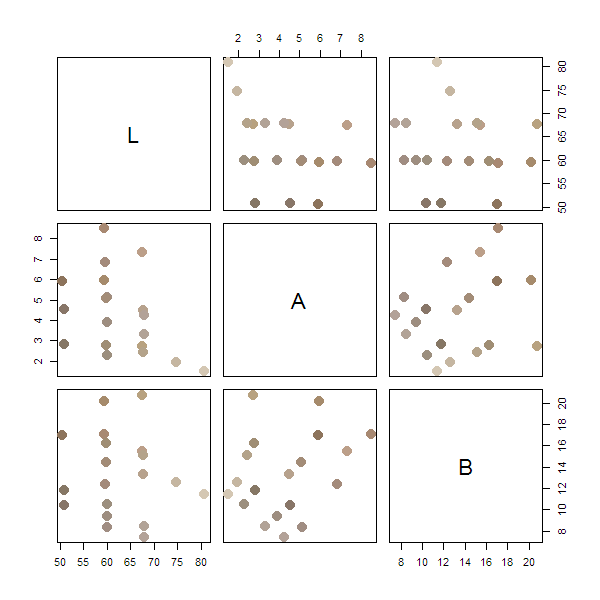[Package aqp version 1.9.1 Index]